# Edexcel A Level Chemistry:复习笔记2.3.4 Halide Ion Reactions

### Halide Ion Reactions

#### Concentrated sulfuric acid

• Chloride, bromide and iodide ions react with concentrated sulfuric acid to produce toxic gases
• These reactions should therefore be carried out in a fume cupboard
• The general reaction of the halide ions with concentrated sulfuric acid is:

H2SO4(l) + X-(aq) → HX(g) + HSO4-(aq)

(general equation)

Where X- is the halide ion

#### Reaction of chloride ions with concentrated sulfuric Acid

• Concentrated sulfuric acid is dropwise added to sodium chloride crystals to produce hydrogen chloride gas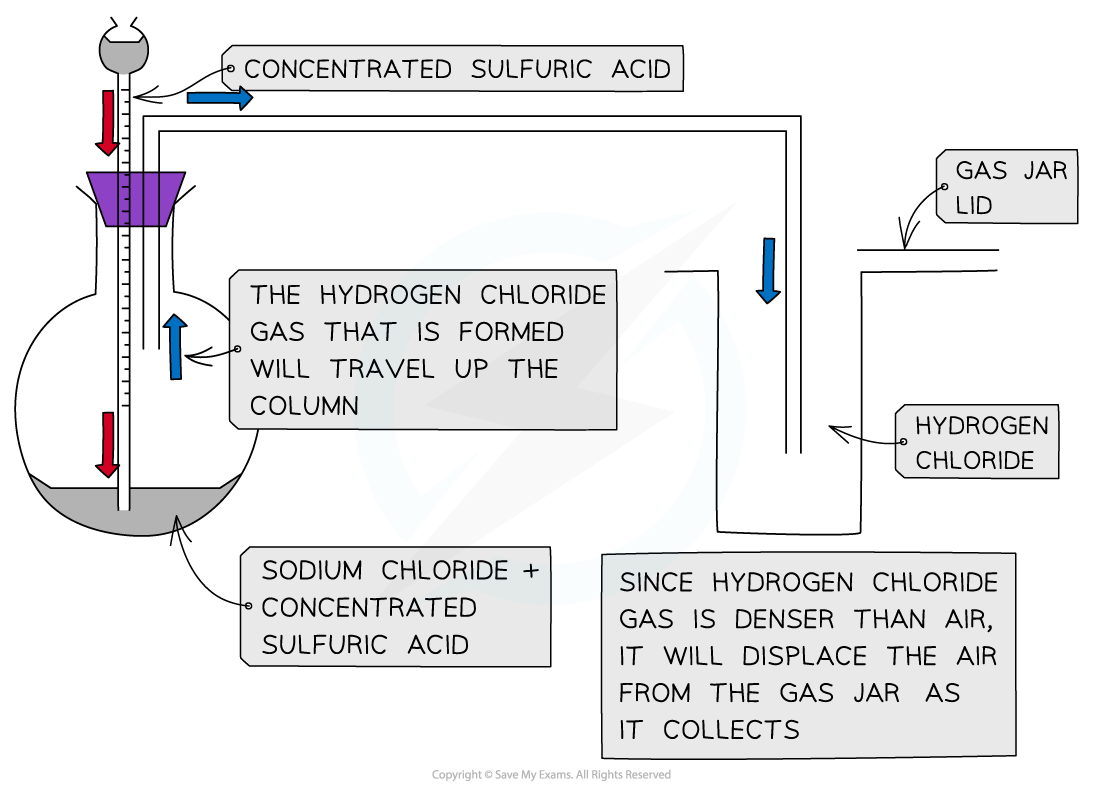Apparatus set up for the reaction of sodium chloride with concentrated sulfuric acid

• The reaction that takes place is:

H2SO4 (l) + NaCl (s) → HCl (g) + NaHSO4 (s)

• The HCl gas produces is seen as white fumes

#### Reaction of bromide ions with concentrated sulfuric acid

• The thermal stability of the hydrogen halides decreases down the group
• The reaction of sodium bromide and concentrated sulfuric acid is:

H2SO4 (l) + NaBr (s) → HBr (g) + NaHSO4 (s)

• The concentrated sulfuric acid oxidises HBr which decomposes into bromine and hydrogen gas and sulfuric acid itself is reduced to sulfur dioxide gas:

2HBr (g) + H2SO4 (l) → Br2 (g) + SO2 (g) + 2H2O (l)

• The bromine is seen as a reddish-brown gas

#### Reaction of iodide ions with concentrated sulfuric acid

• The reaction of sodium iodide and concentrated sulfuric acid is:

H2SO4 (l) + NaI (s) → HI (g) + NaHSO4 (s)

• Hydrogen iodide decomposes the easiest
• Sulfuric acid oxidises the hydrogen iodide to several extents:
• The concentrated sulfuric acid oxidises HI and is itself reduced to sulfur dioxide gas:

2HI (g) + H2SO4 (l) → I2 (g) + SO2 (g) + 2H2O (l)

• Iodine is seen as a violet/purple vapour
• The concentrated sulfuric acid oxidises HI and is itself reduced to sulfur:

6HI (g) + H2SO4 (l) → 3I2 (g) + S (s) + 4H2O (l)

• Sulfur is seen as a yellow solid
• The concentrated sulfuric acid oxidises HI and is itself reduced to hydrogen sulfide:

8HI (g) + H2SO4 (l) → 4I2 (g) + H2S (s) + 4H2O (l)

• Hydrogen sulfide has a strong smell of bad eggs

Halide ion reactions with concentrated sulfuric acid table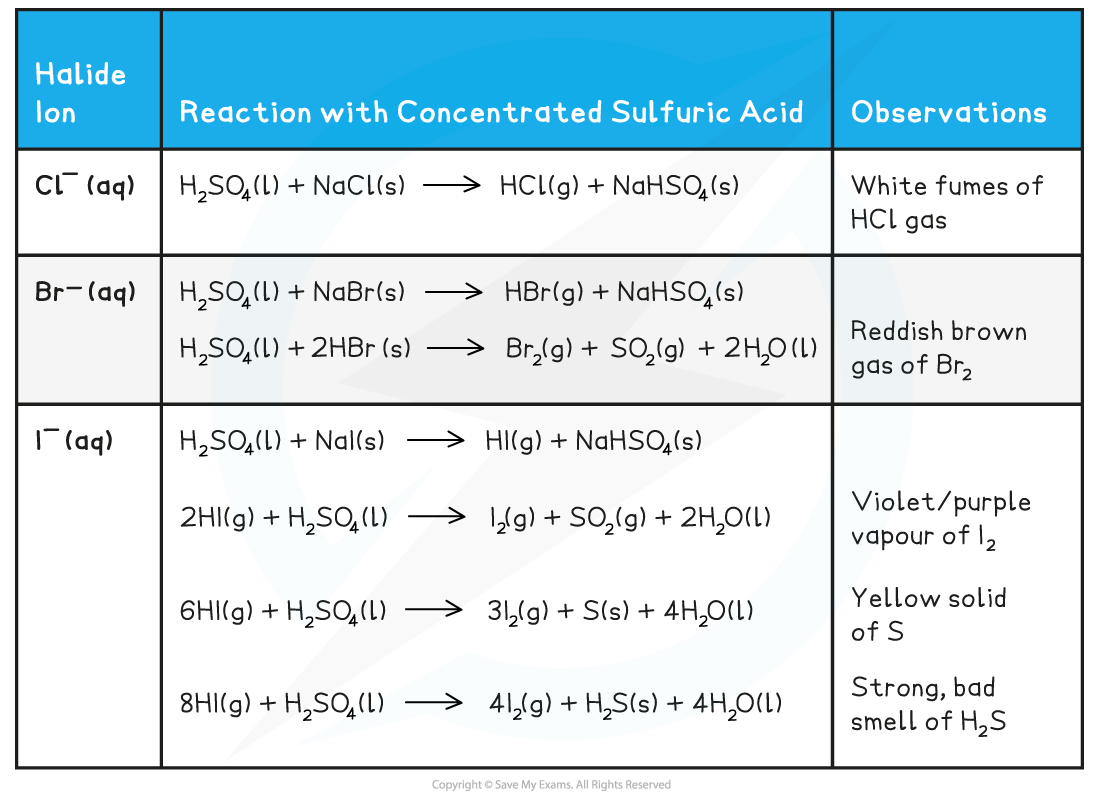#### Silver ions & ammonia

• Halide ions can be identified in an unknown solution by dissolving the solution in nitric acid and then adding a silver nitrate solution followed by ammonia solution
• The halide ions will react with the silver nitrate solution as follows:

AgNO3 (aq) + X- (aq) → AgX (s) + NO3- (aq)

Ag+ (aq) + X- (aq) → AgX (s)

• X- is the halide ion in both equations
• If the unknown solution contains halide ions, then a precipitate of the silver halide will be formed (AgX)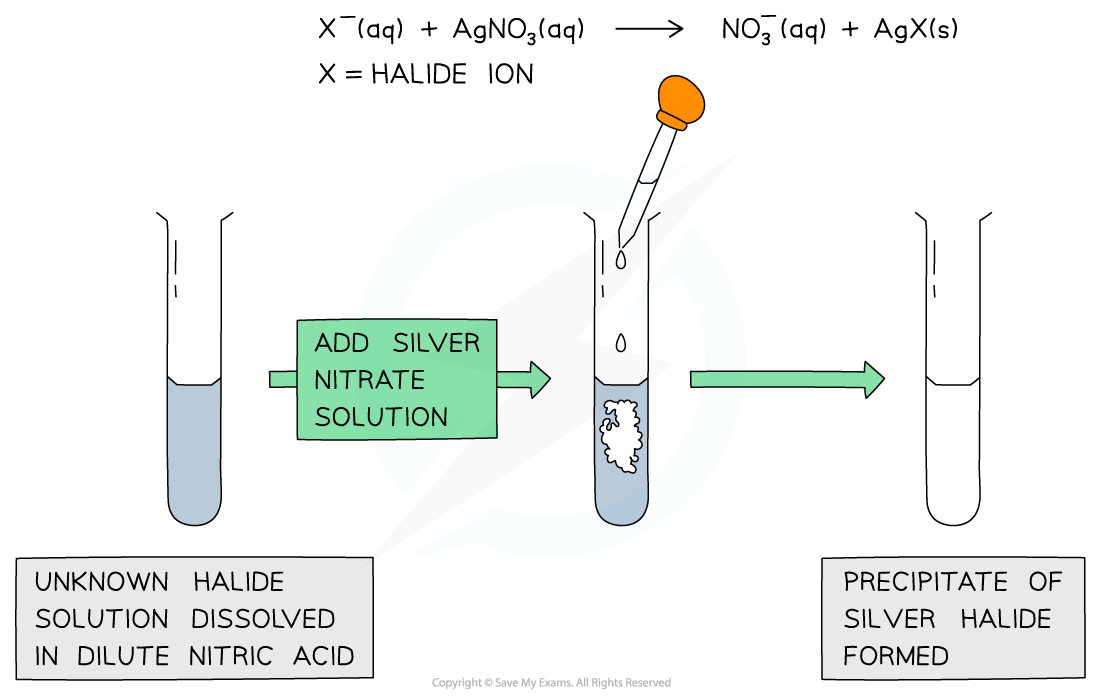A silver halide precipitate is formed upon addition of silver nitrate solution to halide ion solution

• Dilute followed by concentrated ammonia is added to the silver halide solution to identify the halide ion
• If the precipitate dissolves in dilute ammonia the unknown halide is chloride
• If the precipitate does not dissolve in dilute but in concentrated ammonia the unknown halide is bromide
• If the precipitate does not dissolve in dilute nor concentrated ammonia the unknown halide is iodide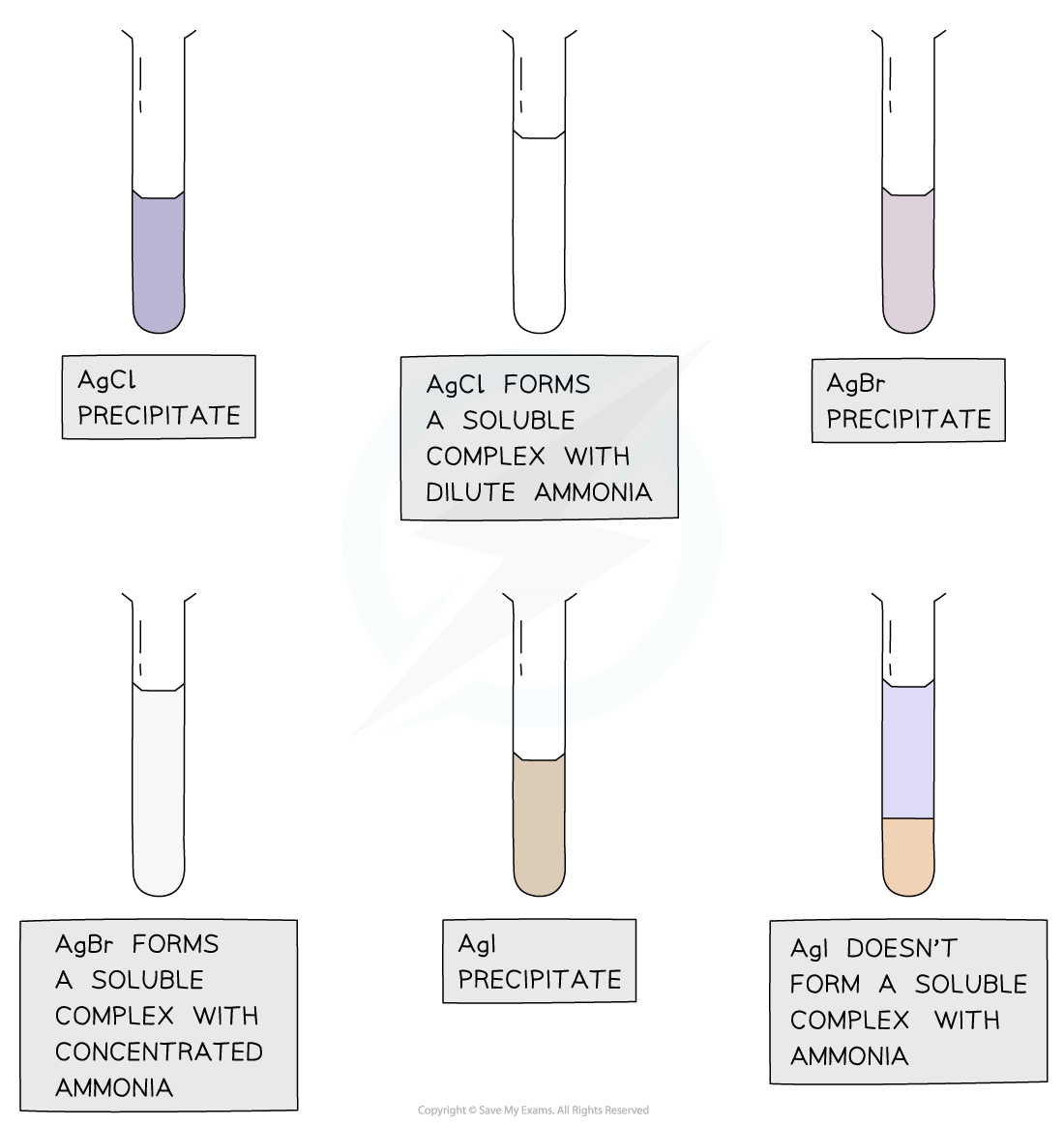Silver chloride and silver bromide precipitates dissolve on addition of ammonia solution whereas silver iodide doesn’t

Reaction of halide ions with silver nitrate & ammonia solutions table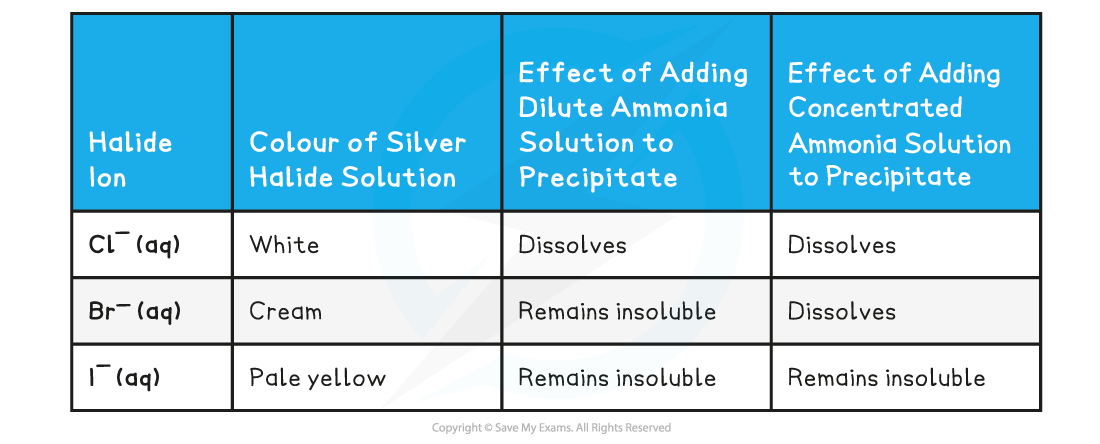#### Reactions with hydrogen halides

• When a halogen reacts with hydrogen a hydrogen halide is produced, for example:
• Cl2 (g) + H2 (g) → 2HCl (g)
• These hydrogen halides react with ammonia gas to form ammonium halides
• NH3 (g) + HCl (g) → NH4Cl (s)
• Hydrogen halides will also react with water
• For example, hydrogen chloride also dissolves in water to form hydrochloric acid
• HCl (g) → H+ (aq) + Cl- (aq)